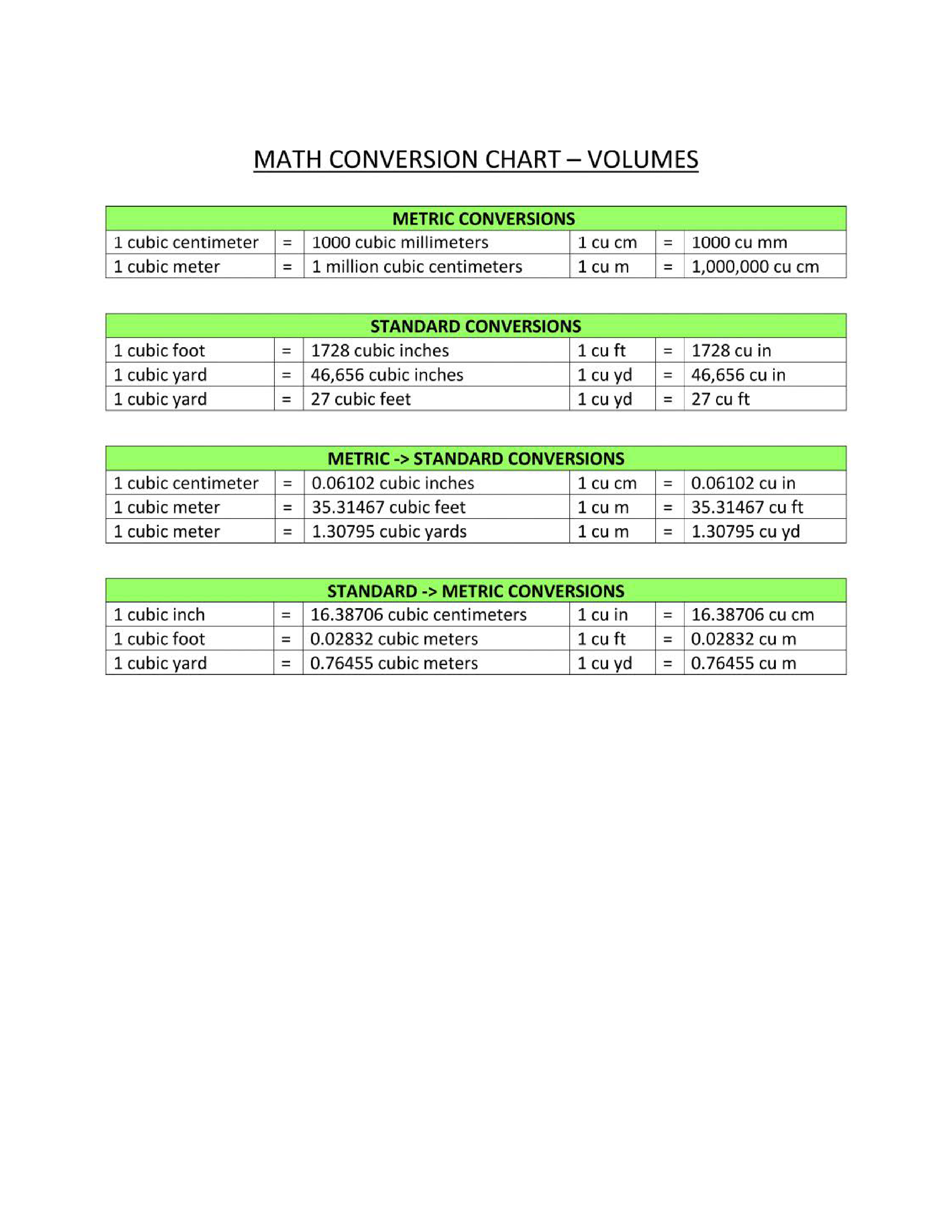# Volume Conversion ChartHaga clic en la imagen para ampliar / Haga clic en el botón de abajo para ver más imágenes

## Guardar, completar los espacios en blanco, imprimir, listo!How to convert volume into different metrics? Download this volume conversion reference chart template that will perfectly suit your needs!

Formatos de archivo disponibles:

.pdf

• Este documento ha sido certificado por un profesionall
• 100% personalizable

Calificación de la plantilla： 7

Malware en virus vrij：Life Privado Education Educación math mates mathematics matemáticas Chemistry Química Conversion Chart Tabla de conversión metric conversion conversión métrica volume conversion chart tabla de conversión de volumen math conversion chart tabla de conversión matemática math conversions chart tabla de conversiones matemáticas conversion chart math tabla de conversión de matemáticas maths conversion table pdf tabla de conversión de matemáticas pdf mathematical conversions list lista de conversiones matematicas mathematical units conversion table pdf tabla de conversión de unidades matemáticas pdf math conversion calculator calculadora de conversión matemática math conversion formulas fórmulas de conversión matemática unit conversion chart printable tabla de conversión de unidades imprimible basic metric conversions chart tabla de conversiones métricas básicas metric conversion chart math templates for kindergarten math templates for teachers math templates free download math themed template math worksheet mathematics certificates mathematics formulas math templates maths templates free microsoft powerpoint templates for mathematics microsoft word math templates powerpoint math templates unit conversion chart pdf unit conversion calculator conversion of units of measurement unit conversion chart physics unit conversion chart chemistry unit conversion chart conversion calculator unit converter unit conversion chart download physics all unit conversion chart unit conversion chart sample unit conversion chart template physics unit conversion chart

How to convert volume into different metrics? Download this volume conversion reference chart template that will perfectly suit your needs!

Often, conversion is needed between units used in the metric system and the imperial system. Therefore, are common online calculators or offline metric conversion charts available. This Metric conversion chart aims to help to convert units of measurement with Metric Converter and Metric Conversion Table into Imperial system units and to better understand alternative measurements that they are unfamiliar with.

For example for Volume Conversion Chart, shows:
• metric volume conversions;
• imperial volume conversions;
• standard volume conversions;
• metric to standard conversions;
• standard to metric conversions.

This Volume Conversion Chart is intuitive, ready-to-use, and structured in a smart way. Try it now and let this mathematics template inspire you. We certainly encourage you to use this Volume Conversion Chart for your own benefit and are confident it will fit your needs

Download this Volume Conversion Chart now and print and place it in sight!

Nada en este sitio se considerará asesoramiento legal y no se establece una relación abogado-cliente.

Deja una respuesta. Si tiene preguntas o comentarios, puede colocarlos a continuación.